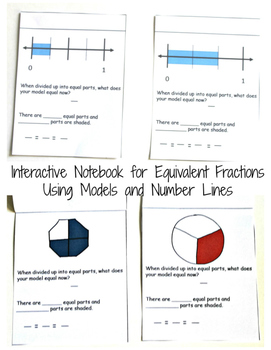# Interactive Notebook for Equivalent Fractions Using Models and Number linesSubject
File Type

PDF

(1 MB|8 pages)
Standards
• Product Description
• StandardsNEW
This printable contains four models to use while teaching equivalent fractions.

Step one: Pass out page one, cut out the four squares, and glue on top of each other.
Step 2: The students figure out fraction for the model.
Step 3: Flip to next page and divide model into equal parts. The students then figure out new numerator and denominator.
Step 4: Repeat step 3, but divide up model differently.
Step 5: Repeat step 3 again but divide up differently again.

Now the students have four fractions that are equivalent.

There are four different models and four different number lines for the students to work on. Eight different fraction in all.

There are four number lines that the students can do the same procedures on.

See http://youvegotthismath.com/2016/05/13/teaching-equivalent-fractions-with-an-interactive-notebook/ for more details on how to use this product.
Explain why a fraction 𝘢/𝘣 is equivalent to a fraction (𝘯 × 𝘢)/(𝘯 × 𝘣) by using visual fraction models, with attention to how the number and size of the parts differ even though the two fractions themselves are the same size. Use this principle to recognize and generate equivalent fractions.
Understand a fraction 1/𝘣 as the quantity formed by 1 part when a whole is partitioned into 𝘣 equal parts; understand a fraction 𝘢/𝑏 as the quantity formed by 𝘢 parts of size 1/𝘣.
Total Pages
8 pages
N/A
Teaching Duration
45 minutes
Report this Resource to TpT
Reported resources will be reviewed by our team. Report this resource to let us know if this resource violates TpT’s content guidelines.